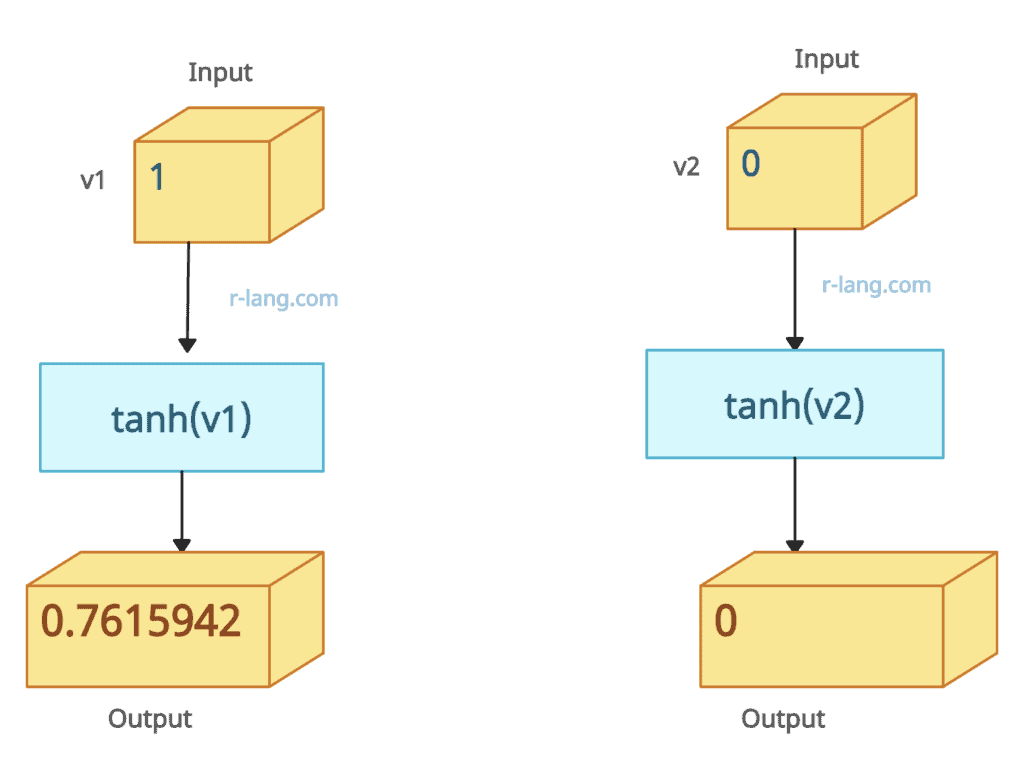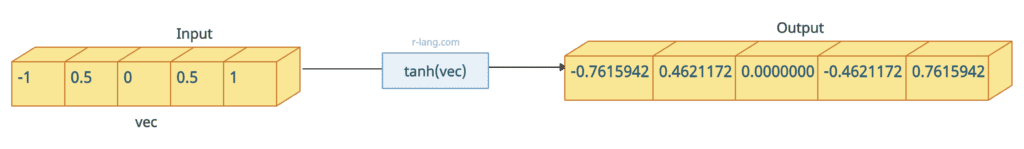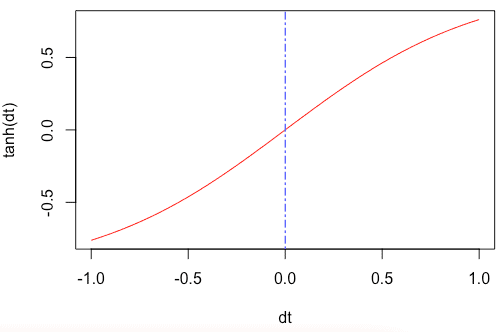# How to Use tanh() Function in R

The tanh() function in R calculates the number’s hyperbolic tangent in radians.

## Syntax

``````tanh(num)
``````

## Parameters

num: It is a numeric value.

## Return value

It returns the hyperbolic tangent of a number (in radians).

## Example 1: Usage of tanh() function``tanh(1)``

Output

`` 0.7615942``

If you pass the 0 to the tanh() function, it will return 0.

``tanh(0)``

Output

`` 0``

## Example 2: Using tanh() function with a vector``````vec <- c(-1, 0.5, 0, 0.5, 1)

tanh(vec)``````

Output

`` -0.7615942 0.4621172 0.0000000 0.4621172 0.7615942``

## Example 3: Passing a pi, positive, and negative number

The pi is a built-in constant; its value is 3.141593.

``````data_one <- tanh(0.9)

data_two <- tanh(-0.75)

data_three <- tanh(0.45) * (180.0 / pi)
print(paste0("tanh(0.45): ", data_three, " degrees"))
``````

Output

`````` "tanh(0.9): 0.716297870199024 radians"
 "tanh(0.45): 24.1730323815932 degrees"
``````

## Plotting the tanh() function

``````dt <- seq(-1, 1, by = 0.05)
plot(dt, tanh(dt), typ = "l", col = "red")
abline(v = 0, lty = 6, col = "blue")``````

OutputThat is it.